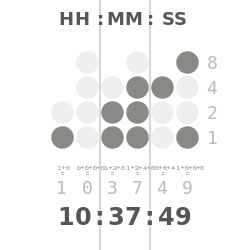## Tuesday, April 7, 2015

### 20140622 Binary Coded Decimal Clock part 1

I decided to use a Ardunio Mega because of more memory and more I/O, even still I think if you removed the LCD this could be used on a UNO with out problems.
A couple of interesting things I found out while reading about the DS1307 Real Time Clock, it’s output is in Binary, one website says that it’s BCD, but it didn’t appear to BCD when I tried it out.
Since it didn’t appear to be BCD, I did have to do a little extra to get a clock to work, it wasn’t hard to do thou.
The RTClib.h library is very clear on how it works. and breaks things out easy like:
now.hour(), now.minute(), now.second(), now.year(), now.month(), now.day()  All of which is straight forward, it give the output “NOW” and it is in binary.
I decided to use one of the 8x8 LED matrix for the clock part of this, decided on using this for a couple of reason, it only uses 3 pins, it has more then enough  LEDs, and the LedControl.h library has a method to send a “on/off” vaule to an entire row or column at once, and that method uses (yup you guessed it) Binary.  SO RTC output is native Binary, the LED Matrix input is native Binary.  BOTH are 8 BIT binary, easy peasy.  Problem, BCD is 4 bit, and while the 8 bit Binary clock worked, it was a lot harder to read, you have to keep up with 8 bits for each hour, minute, and second.  And while it’s true if you split out to BCD you have 3 more digits to deal with you only have 4 BITs per digit (8, 4, 2, 1) so it’s much easier to quickly figure out the time.
and ends up looking like this:So I had to come up with a way to display each digit for the hour, minutes, and seconds -  Since we look at these as decimal numbers (base 10), we have a TENS spot, and a ONES spot to deal with for each (hour, minute, second).
Turns out this was also easy to do, (I’ll admit I found it on one of the arduino forums)
But the formula is very simple:
((now.hour()/10)%10)); Will get the TENS spot and
(0,1, (now.hour()%10)); Will get the ONES spot.
Use this formula for each now.hour, now.minute, now.second
So here is the complete Concept code:
// Date and time functions using a DS1307 RTC connected via I2C and Wire lib
#include <Wire.h>
#include "RTClib.h"
#include "LedControl.h"

RTC_DS1307 RTC;
LedControl lc=LedControl(30,32,34,1);

void setup () {
Serial.begin(9600);
Wire.begin();
RTC.begin();
lc.shutdown(0,false);
lc.setIntensity(0,8);
lc.clearDisplay(0);
if (! RTC.isrunning()) {
Serial.println("RTC is NOT running!");
// following line sets the RTC to the date & time this sketch was compiled
}
}

void loop () {
DateTime now = RTC.now();
Serial.print(now.year(), DEC);
Serial.print('/');
Serial.print(now.month(), DEC);
Serial.print('/');
Serial.print(now.day(), DEC);
Serial.print(' ');
Serial.print(now.hour(), DEC);
lc.setColumn(0,0, ((now.hour()/10)%10));
lc.setColumn(0,1, (now.hour()%10));
colon();
Serial.print(':');
Serial.print(now.minute(), DEC);
lc.setColumn(0,3,((now.minute()/10)%10));
lc.setColumn(0,4,(now.minute()%10));
colon();
Serial.print(':');
Serial.print(now.second(), DEC);
lc.setColumn(0,6,((now.second()/10)%10));
lc.setColumn(0,7,(now.second()%10));
colon();
Serial.println();

}

void colon() {
//    lc.setLed(0,4,2, true);
lc.setLed(0,6,2, true);
// lc.setLed(0,4,5, true);
lc.setLed(0,6,5, true);
delay (250);

// lc.setLed(0,4,2, false);
lc.setLed(0,6,2, false);
// lc.setLed(0,4,5, false);
lc.setLed(0,6,5, false);
delay(250);
}

You can see that it also output the Year, month, day HH:MM:SS to the serial terminal.  All of this is also 24hr time (or military time). This was just the test code, as I will be adding a LCD with button, this will let you change the time if needed, it will also display the time.
So not complete yet.